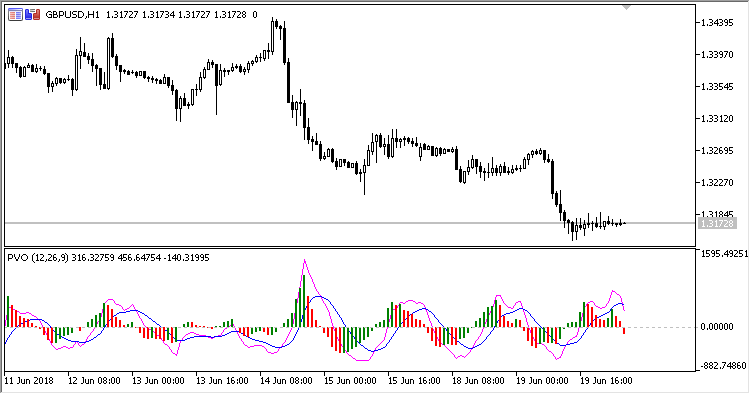Interesting script?
So post a link to it -
let others appraise it

You liked the script? Try it in the MetaTrader 5 terminal# PVO - indicator for MetaTrader 5

Views:
1434
Rating:
Published:
2018.07.13 15:21

Indicator PVO (Percentage Volume Oscillator) represents a momentum for the volume. It calculates the difference in the percents of large MA between two moving averages based on volumes.

It has ten configurable parameters:

• Show histogram - whether to show the histogram (Yes/No);
• Show PVO - whether to show the PVO line (Yes/No);
• Show Signal - whether to show the signal line (Yes/No);
• Fast MA period - fast MA calculation period;
• Fast MA method - fast MA calculation method;
• Slow MA period - slow MA calculation period;
• Slow MA method - slow MA calculation method;
• Signal period - signal line calculation period;
• Signal method - signal line calculation method;
• Calculation mode - calculation mode:
• Absolute - in absolute values;
• Relative - in relative values.

Calculations:

```Signal = MA(PVO, Signal period, Signal method)
Histogram = PVO - Signal
```

Calculation mode Absolute:

`PVO = ShortMA - LongMA`

Calculation mode Relative:

`PVO = (FastMA - SlowMA) / SlowMA`

where:

```FastMA = MA(Volume, Fast MA period, Fast MA method)
SlowMA = MA(Volume, Slow MA period, Slow MA method)
```

Volume - tick volumeTranslated from Russian by MetaQuotes Software Corp.
Original code: https://www.mql5.com/ru/code/21165MPO

Indicator MPO (Midpoint Oscillator) displays price movement without its trend component around its centerpoint. It is displayed as two lines: Price movement line and signal line. Centerline (MidPoint) is zero level of the oscillator.LeManStop

Trend-following indicator LeManStop is a representative of system LeMan and displays Stop Loss levels for the existing positions in the chart. It draws two Stop Loss lines for Long and Short positions, displayed in different colors.RMI

Indicator RMI (Running Median Indicator) is a moving average with the "Running Median" filtering algorithm.SS

Indicator SS (Stochastic Stack) represents a set of eight indicators Stochastic with different parameters, united in a single calculation process.